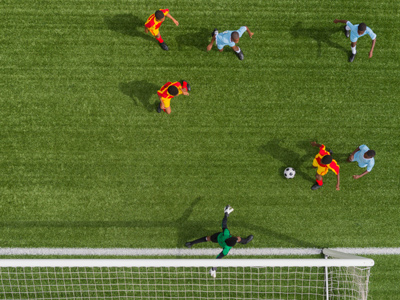An international soccer pitch is 105m x 68m.

# Shapes - Perimeter and Area 1

This Math quiz is called 'Shapes - Perimeter and Area 1' and it has been written by teachers to help you if you are studying the subject at middle school. Playing educational quizzes is a fabulous way to learn if you are in the 6th, 7th or 8th grade - aged 11 to 14.

It costs only \$12.50 per month to play this quiz and over 3,500 others that help you with your school work. You can subscribe on the page at Join Us

In this Math quiz we look at area and perimeter; mainly shapes such as squares and rectangles to give you some easy practice in the subject.

1.
What is the area of a rectangle with a length of 9 cm and a width of 7 cm?
49 cm2
54 cm2
63 cm2
77 cm2
9 x 7 = 63
2.
What is the perimeter of a rectangle with a length of 28 cm and a width of 16 cm
42 cm
64 cm
74 cm
88 cm
3.
To calculate the area of a rectangle it is necessary to multiply the length by the width; how is this formula usually represented?
A = l x w
e = mc2
L = a/w
W = a/l
Area = length x width
4.
If a square has sides that are 13 cm long, what is its area?
26 cm2
52 cm2
105 cm2
169 cm2
5.
A rectangle is 2 meters long and 88 centimeters wide; what is its area?
0.176 meters2
1.76 meters2
3.52 meters2
176 meters2
Don't mix up the meters and centimeters mentioned in the question
6.
Which of the following is not a valid measurement for an area?
102 m2
15 m
18 mm2
52 cm2
Area is always spoken of in terms of 'square' units i.e. 18 square millimeters or 52 square centimeters. In the answers above, 15 m is only a length
7.
A rectangle has a length that is twice its width. If the length is 18 m, what is its perimeter?
36 m
48 m
54 m
72 m
The width of the rectangle must be 9 m
8.
What is the area of a square that has a length of 15 cm and a width of 16 cm?
225 cm2
256 cm2
240 cm2
Not a valid question
If the sides are different sizes then it cannot be a square!
9.
Which of the following is not a 2 dimensional shape?
Rectangle
Sphere
Trapezium
Triangle
A sphere is a 3 dimensional shape. Remember that perimeter and area are only associated with 2 dimensional shapes and therefore you will never be asked to calculate the area or perimeter of a sphere
10.
An international soccer pitch is 105 m x 68 m. What is the area of one half of the pitch?
3,570 meters2
4,624 meters2
7,140 meters2
11,025 meters2
Author:  Frank Evans